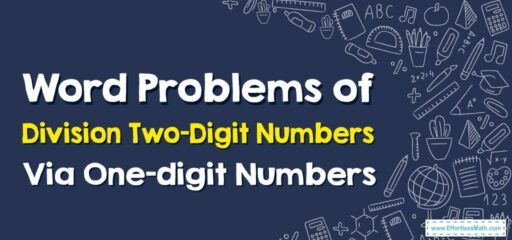# How to Solve Word Problems of Dividing Two-Digit Numbers By One-digit Numbers

Solving word problems involving the division of two-digit numbers by one-digit numbers requires a clear understanding of the problem, identifying the necessary operation, and applying it accurately.## A Step-by-step Guide to Solving Word Problems of Dividing Two-Digit Numbers By One-digit Numbers

Let’s take a look at how to approach word problems involving the division of two-digit numbers by one-digit numbers.

Example Problem: “Lucy has 56 marbles. She wants to distribute them equally into 8 bags. How many marbles will be in each bag?”

### Step 1: Understand the Problem

First, make sure you understand the problem. Read it carefully. In this problem, Lucy has 56 marbles and she wants to distribute them equally into 8 bags. We need to find out how many marbles each bag will contain.

### Step 2: Identify the Operation

Next, identify the operation you need to perform to solve the problem. In this case, since Lucy is distributing marbles equally, we know we need to use division.

### Step 3: Set up the Division Problem

The total number of marbles (56) is the dividend, and the number of bags (8) is the divisor. Set up the problem as $$56÷8$$.

### Step 4: Solve the Division Problem

Perform the division operation. 56 divided by 8 equals 7.

### Step 5: Interpret the Answer

The answer to the division problem tells you the number of marbles per bag. So, each bag will contain 7 marbles.

### Step 6: Check Your Work

Finally, you can check your work by multiplying your answer by the divisor. In this case, 7 (marbles per bag) times 8 (bags) equals 56 (total marbles), which matches the original problem.

So, the final answer to the problem is: “Each bag will contain 7 marbles.”

Remember to always read the problem carefully, identify the correct operation, and check your work at the end. Practice will help you get better at solving these kinds of problems!

### What people say about "How to Solve Word Problems of Dividing Two-Digit Numbers By One-digit Numbers - Effortless Math: We Help Students Learn to LOVE Mathematics"?

No one replied yet.

X
30% OFF

Limited time only!

Save Over 30%

SAVE $5 It was$16.99 now it is \$11.99